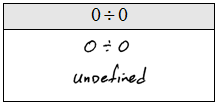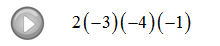## Pages

### Multiplying and Dividing Integers

YouTube Playlist on Real Number Operations

Multiplying a negative number by a positive number will result in a negative number. And when multiplying two negative numbers the result will be positive. Be careful when simplifying 5(−6), the operation here is multiplication NOT subtraction so 5(−6) = −30. The rules for division are the same.

(Negative) x (Positive) = (Negative)
Example: (−7)(+4) = −28

(Negative) x (Negative) = (Positive)
Example: (−7)(−4) = +28

Also, zero times anything is zero.

Multiply or Divide.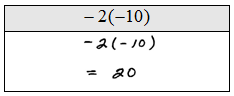Dividing by zero is undefined. What happens when you try to divide by zero on a calculator?

Zero and Division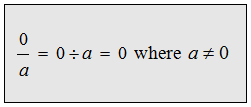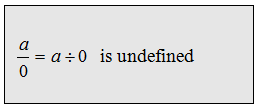Divide.